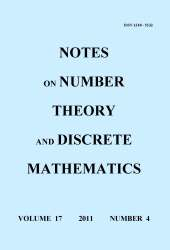# Notes on Number Theory and Discrete Mathematics

### ISSN: 1310-5132International Journal Notes on Number Theory and Discrete Mathematics is specialized in the area of number theory and discrete mathematics. The main topics of research, covered by the Journal, are arithmetic functions, prime numbers, Fibonacci sequence and its generalizations, Diophantine equations, combinatorics, combinatorial identities and inequalities, discrete mathematics, and others.

The Journal is refereed by Zentralblatt MATH.

Editorial office